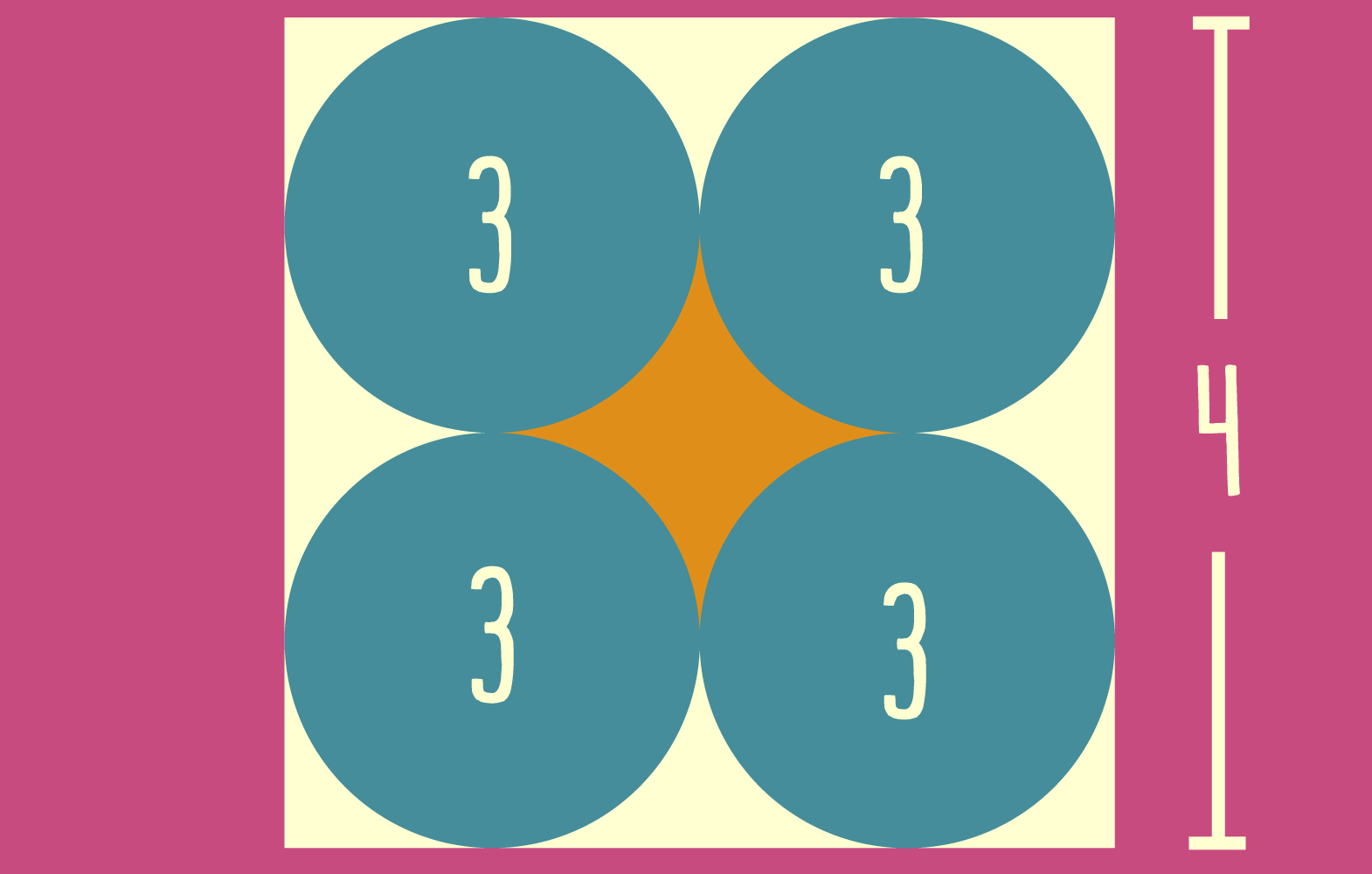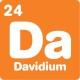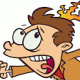# Blog

## Circle gaps brainteaser

By

,

What is the area of the orange star in the centre? The blue circles each have an area of 3 square centimetres, and the big square has sides that are 4 centimetres long.Scroll down or click for a hint, or the answer!## Brainteaser hint

A good place to start is calculating the area of the big square. You can subtract the areas of the circles, and then try to work out what to do with what’s left!There are lots of strange shapes in this puzzle! But with a bit of smart thinking, we can put them together.

We can start by calculating the area of the big square: 4 x 4 = 16 square centimetres.

Then, we can subtract the areas of the circles: 16 – 3 – 3 – 3 – 3 = 4 square centimetres.

What do we have left? We have the diamond in the middle, but we also have some other scraps too. There’s a rounded triangle shape on the top, bottom, left and right sides, and a smaller shape in each corner.

The key is combining these shapes. If we take the rounded triangles from the left and right sides and put them together, we get exactly the same diamond shape as in the middle!

We can do the same with the top and bottom rounded triangles.

And the 4 shapes in the corners will come together to make yet another diamond shape.

All the scraps together have an area of 4 square centimetres, and we can make exactly 4 diamond shapes using all the scraps. So each diamond must have an area of 1 square centimetre!

Categories:

## 5 responses

1.Peter Perry

Of course, to touch the edges, the circles would need to have an area of 3.141592653… sq cm. As stated, there would be a gap of less than 0.05 cm between circles and edges, and about 0.09 cm between circles. If circles actually touch, then calculation becomes a little more complex, but still doable by considering the square made by joining circle centres.

1.David Shaw

Yup! Given the target demographic for these puzzles, I thought rounding the areas was the best course of action. The brainteaser initially only had the side length of the square, but the circle area formula is a bit hard for many of our readers.

2.David Evans

I just cut the square up into quarters.
Each quarter was 2×2 = 4. Each quarter had 1 circle = 3.
Therefore the four equal parts (Scraps) around the circle had a combined area of 1. (4-3) = 1.
As the scrap from the middle star was one 1/4 of the star. The star had to have an area = 1.

1.David Shaw

That’s a great way to solve the question, David!

3.Missy Lee

Love this one!

This site uses Akismet to reduce spam. Learn how your comment data is processed.

By posting a comment you are agreeing to the Double Helix commenting guidelines.